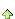共有588人关注过本帖树形打印复制链接

# 主题：易表最新所得税计算方法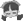xds950802
1楼 | 信息 | 搜索 | 邮箱 | 主页 | UC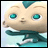加好友发短信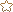下载信息  [文件大小：   下载次数： ]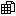点击浏览该文件:易表最新个人所得税计算方法.egd

if([实发工资] <= 5000,0,if(([实发工资]-5000) <36000,( [实发工资]-5000)*0.03,if(([实发工资]-5000) < 144000,( [实发工资]-5000)*0.1-2520,if(([实发工资]-5000) < 300000,( [实发工资]-5000)*0.2-16920,if(([实发工资]-5000) < 420000,( [实发工资]-5000)*0.25-31920,if(([实发工资]-5000) < 660000,( [实发工资]-5000)*0.3-52920,if(([实发工资]-5000) < 960000,( [实发工资]-5000)*0.35-85920,([实发工资]-5000)*0.45-181920)))))))